﻿ Review of Circles (and related concepts)

# REVIEW OF CIRCLES (and related concepts)

• PRACTICE (online exercises and printable worksheets)

## DEFINITION OF CIRCLE

 By definition, a circle is the set of all points in a plane that are the same distance from a fixed point (called its center). Notes: Let $\,C := (h,k)\,$ be a point; let $\,r\,$ be a nonnegative real number. Using set-builder notation: \begin{align} &\text{the circle determined by \,C\, and \,r\,}\cr\cr &\qquad =\{\ (x,y)\ |\ \text{the distance from (x,y) to (h,k) is r } \ \} \cr\cr &\qquad = \{\ (x,y)\ |\ \sqrt{(x-h)^2 + (y-k)^2} = r\ \}\cr\cr &\qquad = \{\ (x,y)\ |\ (x-h)^2 + (y-k)^2 = r^2\ \} \end{align} If $\,r = 0\,$, the circle degenerates to a single point—its center. In this case, it is called a ‘point circle’. The interior of the circle with center $\,(h,k)\,$ and radius $\,r\,$ is: $$\{(x,y)\ |\ (x-h)^2 + (y-k)^2 < r\}$$ A circle does not include its interior; it is the boundary (of the interior) only. In particular, a (non-point) circle does not include its center. See Equations of Circles for more information.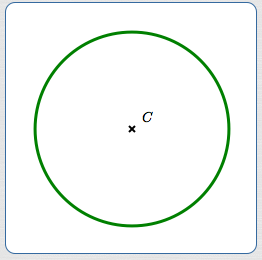a circle (in green) with center $\,C\,$

Depending on context, radius can mean:
1. the distance from the center to any point on the circle
2. a line segment from the center to a point on the circle
Note that the length of a radius (as in 2) is the radius of the circle (as in 1).

The plural of ‘radius’ is either:radius ($\,r\,$) as a distance(a nonnegative number)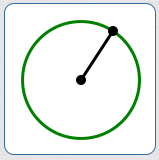radius as a line segment

## DIAMETER OF A CIRCLE

Depending on context, diameter can mean:
1. twice the radius (a nonnegative number)
2. a line segment passing through the center of a circle,
with both endpoints on the circle
Note that the length of a diameter (as in 2) is the diameter of the circle (as in 1).
• In algebra, precalculus, calculus, and life (!) contexts, the words radius and diameter typically refer to numbers (distances).
• In geometry contexts, the words radius and diameter often refer to line segments.
• From context, it should always be clear whether the words radius and diameter refer to numbers (possibly with units) or line segments.diameter ($\,2r\,$) as a distance(a nonnegative number)diameter as a line segment

## CIRCUMFERENCE OF A CIRCLE

 The circumference of a circle is the distance around the circle. As discussed below: $$\text{circumference} = 2\pi r = \pi d\ ,$$ where $\,r\,$ is the radius and $\,d = 2r\,$ is the diameter.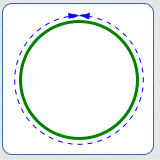circumference of a circle: the distance around the circle

## THE IRRATIONAL NUMBER PI

 Compute with circles, and you're certain to run into the special number $\,\pi\$: SYMBOL USED FOR PI: The symbol ‘$\,\pi\,$’ is a lowercase letter in the Greek alphabet. It is spelled ‘pi’ (no ‘e’ on the end). It is pronounced ‘pie’ (as in apple pie or cherry pie). HANDS-ON UNDERSTANDING OF $\,\pi\,$: Grab any circular object (like a plate). Measure its circumference and diameter. (Use a string or a flexible ruler.) Be sure to use the same units for both measurements. Take the circumference and divide by the diameter—you'll get a number a bit more than $\,3\,$! Repeat with a different circular object, then another (until bored). Any differences in your calculations—as you use different size circles—are due only to measurement errors. DEFINITION OF PI: For circles, the ratio of circumference to diameter is always the same! This was known by Babylonians and Egyptians at least 4000 years ago. By definition, $\,\pi\,$ is the ratio of circumference to diameter in a circle. Leonhard Euler began the use of the symbol ‘$\,\pi\,$’ for this ratio in 1737. $$\pi = \frac{\text{circumference}}{\text{diameter}}$$ The formula for the circumference of a circle follows immediately: $$\text{circumference} = \pi(\text{diameter})$$ PI IS IRRATIONAL: The number $\,\pi\,$ is irrational (not rational). This means: it has no representation as a ratio of integers; a common rational approximation is $\displaystyle\,\pi\approx\frac{22}{7}\,$ it has an infinite, non-repeating decimal expansion; a common decimal approximation is $\,\pi\approx 3.14\,$ for more decimal places of pi, look to the right, or go to Wolfram|Alpha and type in ‘pi’ PRACTICAL INTERPRETATION OF PI: Keep in mind what all this is saying! In any circle, if you walk all the way around, you'll travel just over three times further than if you walk straight across (passing through the center) to the opposite side.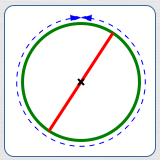By definition, $\,\pi\,$ is the ratio of circumference to diameter in a circle: $$\pi := \frac{\color{blue}{\text{circumference}}}{\color{red}{\text{diameter}}} \approx 3.14$$Thus, $\text{circumference} \approx (3.14)(\text{diameter})$ It takes just over $\,3\,$ diameters to go around the circle! $\pi\,$ is irrational, hence has an infinite, non-repeating decimal expansion $\pi\,$ is approximately 3.1415926535897932384626433832795028841971693993751058209 749445923078164062862089986280348253421170679821480865132 823066470938446095505822317253594081284811174502841027019 385211055596446229489549303819644288109756659334461284756 482337867831652712019091456485669234603486104543266482133 936072602491412737245870066063155881748815209209628292540 917153643678925903600113305305488204665213841469519415116 094330572703657595919530921861173819326117931051185480744 623799627495673518857527248912279381830119491298336733624

## CENTRAL ANGLE OF A CIRCLE

By definition, a central angle in a circle is an angle formed at the center of a circle by two radii.

Notes on central angles:
• By convention, ‘central angle’ refers to the smaller of the two angles determined by two radii.
• That is—unless otherwise requested—a central angle is between
$\,0^\circ\,$ and $\,180^\circ\,$.
• If you want the bigger central angle, ask for the ‘reflex central angle’.
• The name ‘central angle’ is appropriate, since the vertex of a central angle is the center of a circle.
The sizes of angles ($\,\theta\,$) are often described using these words:
 the zero angle equal to zero $\,\theta = 0^\circ\,$ acute strictly between $\,0^\circ\,$ and $\,90^\circ\,$ $\,0^\circ < \theta < 90^\circ\,$ right equal to $\,90^\circ$ $\theta = 90^\circ$ obtuse strictly between $\,90^\circ\,$ and $\,180^\circ\,$ $\,90^\circ < \theta < 180^\circ\,$ straight equal to $\,180^\circ$ $\theta = 180^\circ$ reflex strictly between $\,180^\circ\,$ and $\,360^\circ\,$ $180^\circ < \theta < 360^\circ$a central angle (the smaller angle determined by two radii)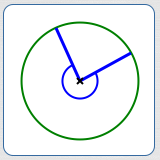a reflex central angle (the bigger angle determined by two radii)

Master the ideas from this section